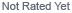Loading... Please wait...
• My Account

Currency Displayed in

Resistor Values & Units

Resistor values are in ohms with symbol . Values indicated as Kohms or Kor kiloohms are in thousands of ohms. Values indicated as Mohms or Mor megohms are in millions of ohms.

To convert Kto M, multiply by .001

Example: 220K= .220M= 220,000

To convert Mto K, multiply by 1,000

Example: 3.3M= 3300K= 3,300,000

NOTE: Unfortunately some pieces of vintage equipment used “M” to indicate thousands of ohms instead of “K” on schematics and parts lists. Typically these would not use or mix “K” and “M”. Best bet is to carefully check before replacing, etc. Since most equipment did not use resistors above 20M, if values are listed above 20M, it may be a good indicator that the “M” reference is actually to KΩ.

Resistor Fundamentals

“Ohm’s Law” governs the application of resistors in a basic circuit. Ohm’s Law is the simple formula:

E = I x R, where

I is current thru the resistor in amperes,

E is the voltage across the resistor in volts,

R is the resistance in ohms ().

Simple re-arrangement of Ohm’s Law, also yields:

I = E/R or R = E/I

The power dissipation of a resistor may be calculated using the formula:

P= E x I, where

P is the power dissipation in watts,

E is the voltage across the resistor in volts,

I is current thru the resistor in amperes.

## New Products

•••••